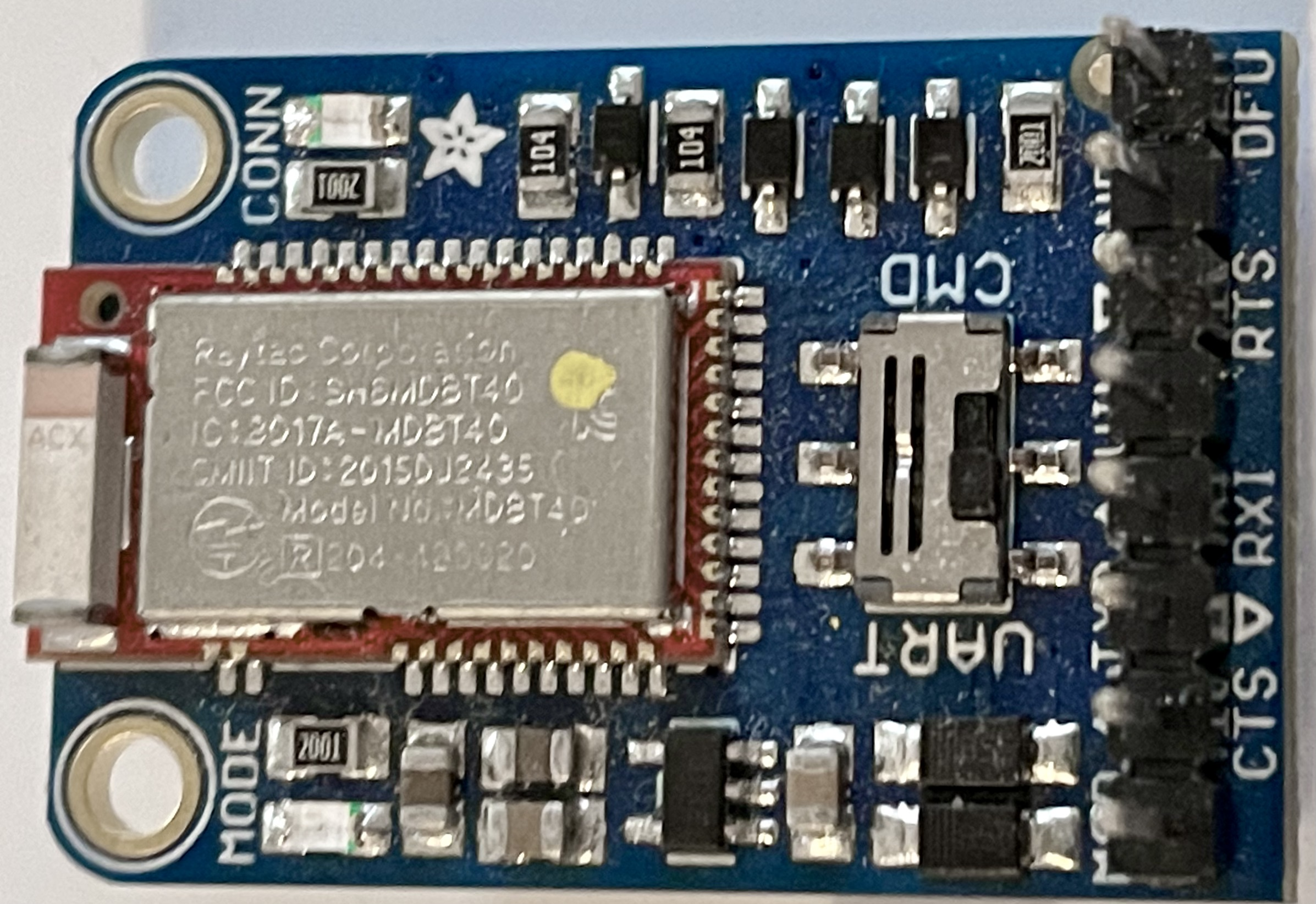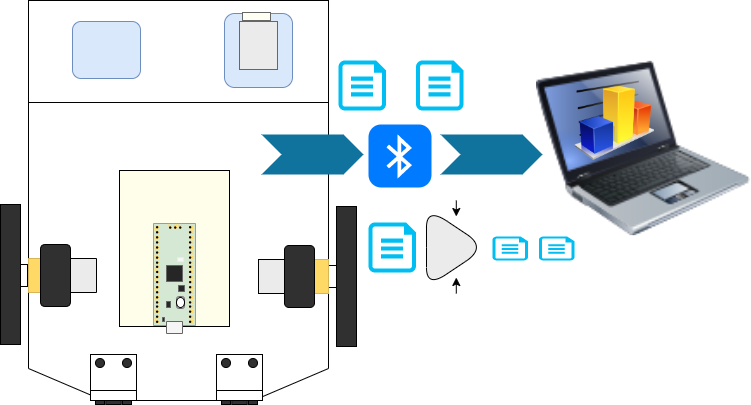A Bluefruit BLE UART Friend (Bluetooth BLE) can add Bluetooth BLE to a project, with support in a Bluefruit Connect App to visualise data. Ulab is an implementation of parts of Numpy and SciPy for microcontroller Python interpreters, like CircuitPython and Micropython. This project uses CircuitPython, but similar issues would be seen in Micropython.

My use case involves largish uLab arrays of floating point data to send, which are then visualised in a Bleak based desktop app. This is very slow however, due to the slow 9600 baud rate of this UART.The baud rate could be increased, but having already seen some errors across this UART using simple Dupont leads, I didn’t want to push my luck. An answer could be to buy the SPI Bluefruit BLE Friend, which might be faster, but that is spending more money on a device I’ve already bought and integrated. Another might be to swap this design for the Raspberry Pi Pico W, although there may be a memory sacrificed for the Bluetooth stack there.You can build this autonomous low-cost Raspberry Pi Pico robot yourself using Robotics at Home with Raspberry Pi Pico!

So the other answer is to see what I can fix in software. I can probably use fewer bytes to send the same data, with savings that might be quite significant.

What I want to check was the overheads, in complexity, and size of sending base64 encoded bytes, instead of a JSON list.

## The JSON List

Sending as JSON has a clear advantage in readability, I can easily glance at debug data, although for large lists that is less useful and I intend to let code consume the data received.

## Base64 encoding

Numpy (or uLab) arrays can be converted to and from a binary bytes representation. This representation will be the array header data plus the raw array buffer. The bytes representation is efficient.

Base64 encodes this back into string data, effectively using 64 printable characters. These are the lowercase letters, uppercase letter, numbers 0-9 and the ‘+’ and ‘/’ characters.

`aGVsbG8K` is the base64 encoded version of `hello\n`.

## Comparing their code

Lets see the code to check both types using a CircuitPython REPL via serial.

``````>>> from ulab import numpy as np
>>> import random
>>> import json
>>> import binascii``````

Next, I set up a test subject array using random data:

``````>>> d = np.array([random.uniform(0, 1) for n in range(200)], dtype=np.float)
>>> d
array([0.587398, 0.72429, 0.226611, ..., 0.515096, 0.333402, 0.378693], dtype=float32)``````

This is 200 items of floating point data between 0 and 1. Floating point numbers use 4 bytes per item.

We can now see the size of this list in JSON as an array:

``````>>> len(json.dumps(d.tolist()))
2003
>>> json.dumps(d.tolist())
'[0.587398, 0.72429, 0.226611,...
0.515096, 0.333402, 0.378693]'``````

I’ve abridged the output as it is around 2Kb. It converts the `ndarray` to a standard python list, then dumps it.

Let’s try with `tobytes`.

``````>>> len(json.dumps(
...   binascii.b2a_base64(d.tobytes())
... ))
1072``````

This bytes version is around half the size, with a little overhead. It’s wrapped in JSON again, so you could have other metadata with it if needed. We have to convert the np array to bytes, then to base64, then this would be wrapped in our json data.

A 16 bit integer would use 2 bytes, but in JSON could be 5 characters, which along with removing the list representation may only need around 40% of the space.

Setting up the list:

``````>>> d = np.array([int(random.uniform(0, 65535)) for n in range(200)], dtype=np.uint16)
>>> d
array([34024, 3334, 44281, ..., 2270, 64406, 56325], dtype=uint16)``````

Trying the json:

``````>>> len(json.dumps(d.tolist()))
1369
>>> json.dumps(d.tolist())
'[34024, 3334, 44281, ... ]'``````

And in base64:

``````>>> len(json.dumps(
...   binascii.b2a_base64(d.tobytes())
... ))
540
>>> json.dumps(
... binascii.b2a_base64(d.tobytes())
... )
'"6IQGDf
...==\\n"'``````

At 540 bytes, it is pretty close to 40% and still worthwhile if size is the primary concern. I’ve abridged the output data.

## Converting it back

None of these tests show me retrieving values back on the computer Python Numpy. Does this work?

Going to try with uint16 first, then the float.

### Uint16 converting back

Lets try this on a python3, assuming Numpy is installed:

``````>>> import numpy as np
>>> import json
>>> import base64
>>> data = '"6IQGDf
...==\\n"'``````

We’ve got the imports ready. Let’s make a conversion:

``````>>> raw_bytes = base64.b64decode(json.loads(data))
>>> d = np.frombuffer(raw_bytes, dtype=np.uint16)
>>> d
array([34024,  3334, 44281 ... ])``````

Yes! These values are the right values. A float might be trickier.

Let’s get a fresh set of floats on the CircuitPython side:

``````>>> d = np.array([random.uniform(0, 1) for n in range(200)], dtype=np.float)
>>> d
array([0.295353, 0.967459, 0.759637, ..., 0.217624, 0.99465, 0.875593], dtype=float32)``````

Now encode it:

``````... binascii.b2a_base64(d.tobytes())
... )
'"jDiXPmirdz+Yd..."'``````

``````>>> data = '"jDiXPmirdz+Yd..."'
>>> d = np.frombuffer(raw_bytes, dtype=np.float32)
>>> d
array([0.2953533 , 0.9674592 , 0.75963736 ... ])``````

It looks good. Note that the PC is displaying a few more significant figures, but it’s the same data.

## Further investigations to try

I should investigate with 2 dimensional arrays and see how those differ. Loading them might be trickier as this assumes 1 dimension in from buffer.

## Robotics at Home with Raspberry Pi Pico

This post was based on research I did for the book Robotics at Home with Raspberry Pi Pico which is available now.